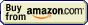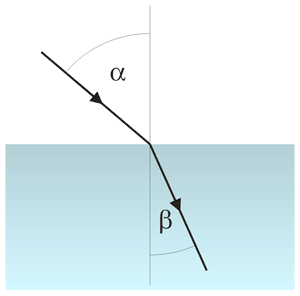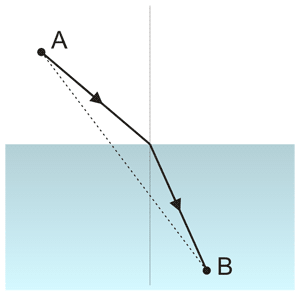# Refractometry## Refractometry » Refraction

In general, light travels in straight lines, but there are some specific circumstances in which light path can become more complicated. First of all, light can get reflected from a mirror - while path before and after reflection is straight, during reflection light changes its direction. There is also other important case in which light changes its direction and doesn't follow straight line - that's when the light gets refracted. Light travels at different speeds in different media - it is fastest in vacuum, but it slows down in gases and even more in liquids and solids. As long as light maintains the same speed (and is not reflected) it travels in straight lines. However, when the speed changes, light direction can change too. Refraction is most often observed when light crosses phase boundary between two different media, like air and water, but it can be also seen whenever light passes through the mixture of gases of different temperatures (which means different densities). That's why images seen through the air over hot objects (hot car, fireplace) seem to be moving. In fact the same happens with other waves, but we will concentrate on the light.

Light speed depends on properties of the medium. In vacuum light travels at the maximum speed (famous c=299,792,458 m/s). For the same medium increasing its density (compressing gas, increasing concentration of solution) slows the light. When comparing different media this dependence between light speed abd density doesn't have to be true - for example speed of light in the densest types of glass (d=8.0 g/cm3) is around 158×106m/s, while in diamond (d=3.5 g/cm3) it is around 124×106m/s, so even if the glass is over two times more dense, speed of the light in the glass is substantially higher than in the less dense diamond. (It is worth of noting here that light speed is also - in most media, but importantly not in vacuum - function of the wavelength.)

Light refraction is a simple consequence of Fermat's principle, which states that light travels on such paths that the time needed to move from one point to another is always the shortest one. Fermat's principle is also called the principle of the least time.

Light refraction is quantitaively described by the Snell's law. Before we will get to the Snell's law we need to define angles involved in refraction.

There are two angles that describe the refraction - angle of incidence, and angle of refraction. They are both measured between the light and a line perpendicular (normal) to the surface. On the picture below angle of incidence is marked α, and angle of the refraction is marked as β.Light refraction - incident (α) and refracted (β) rays.

When the light is almost parallel to the surface, it is sometimes easier to use not an incident angle, but angle between surface and ray, we call it a grazing angle. Sum of the grazing angle and incident angle is 90 degrees. Incidence at such small angles can be referred to as grazing incidence.

In the medium with variable density, where there is no well defined surface between two objects, angle is measured between direction of the light and the density gradient vector.

In an isotropic media light speed is always constant, so light travels in straight lines, which are the shortest paths between any two given points. At constant speed shortest path means also the shortest time of travel, so the straight line satisfies the Fermat's principle. However, if the light has to travel in two different media, and with a different speed in each one, the shortest path no longer guarantees the shortest time.

Let's take a look at the path followed by the light moving from point A to point B:Light refraction - the shortest path and the shortest travel path.

The shortest path is marked with a dotted line. While the path is short, light has to travel relatively long through the medium in which it travels slowly, which makes total time unnecesarilly long. Traveling longer path with higher speed, and shorter path with lower speed makes total travel time shorter. Thus, to satisfy Fermat's principle, light travels the path marked with solid line. Exact angles depend on the relative speeds of the light in both media.

Refraction occurs not only for light, but also for any other waves travelling through any other media.

This site is in construction. More to come. Page was last modified on March 17 2011, 00:00:00.